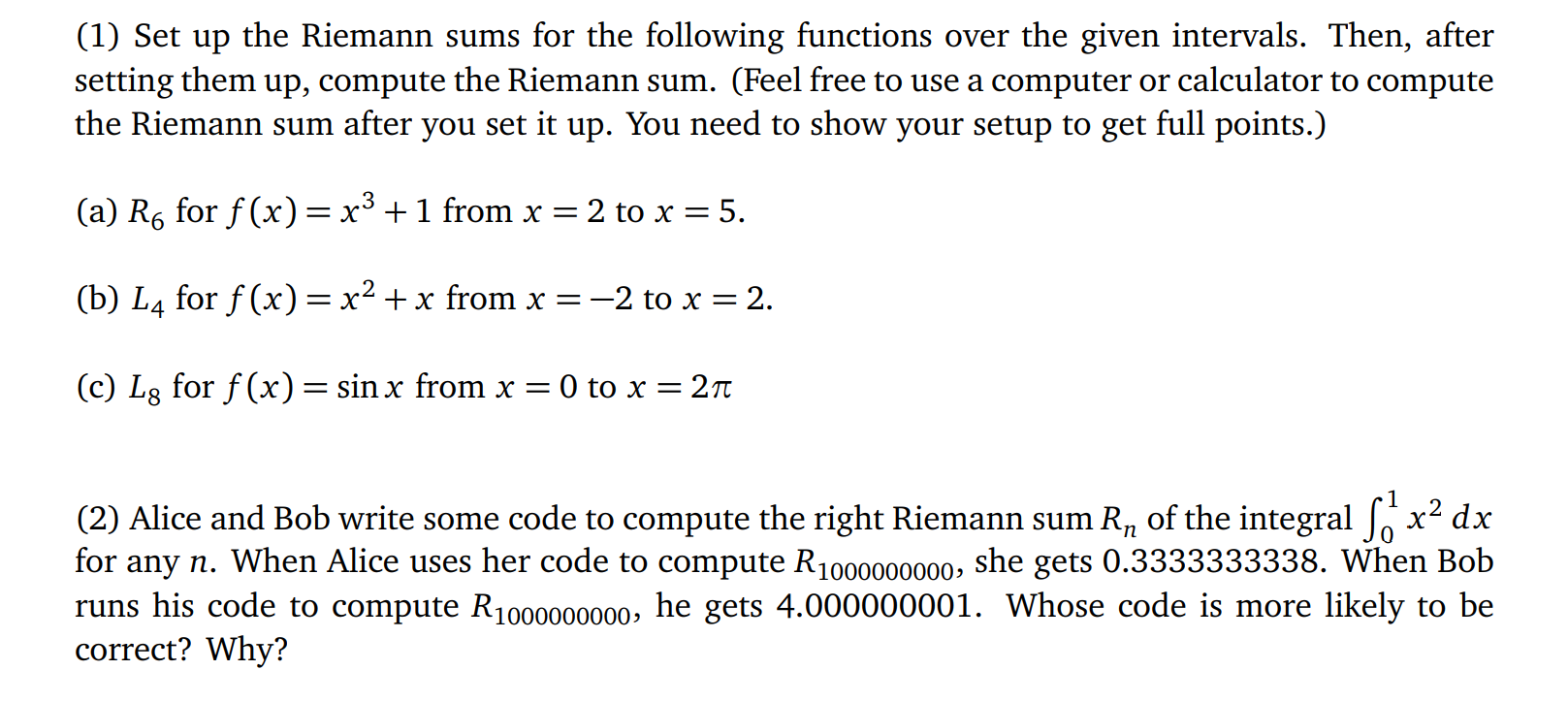Home / Expert Answers / Calculus / 1-set-up-the-riemann-sums-for-the-following-functions-over-the-given-intervals-then-after-sett-pa428

# (Solved): (1) Set up the Riemann sums for the following functions over the given intervals. Then, after sett ...(1) Set up the Riemann sums for the following functions over the given intervals. Then, after setting them up, compute the Riemann sum. (Feel free to use a computer or calculator to compute the Riemann sum after you set it up. You need to show your setup to get full points.) (a) $$R_{6}$$ for $$f(x)=x^{3}+1$$ from $$x=2$$ to $$x=5$$. (b) $$L_{4}$$ for $$f(x)=x^{2}+x$$ from $$x=-2$$ to $$x=2$$. (c) $$L_{8}$$ for $$f(x)=\sin x$$ from $$x=0$$ to $$x=2 \pi$$ (2) Alice and Bob write some code to compute the right Riemann sum $$R_{n}$$ of the integral $$\int_{0}^{1} x^{2} d x$$ for any $$n$$. When Alice uses her code to compute $$R_{1000000000}$$, she gets $$0.333333333$$. When Bob runs his code to compute $$R_{1000000000}$$, he gets $$4.000000001$$. Whose code is more likely to be correct? Why?

We have an Answer from Expert